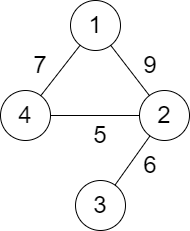2492. Minimum Score of a Path Between Two Cities
Medium
1.6K
272

You are given a positive integer `n` representing `n` cities numbered from `1` to `n`. You are also given a 2D array `roads` where `roads[i] = [ai, bi, distancei]` indicates that there is a bidirectional road between cities `ai` and `bi` with a distance equal to `distancei`. The cities graph is not necessarily connected.

The score of a path between two cities is defined as the minimum distance of a road in this path.

Return the minimum possible score of a path between cities `1` and `n`.

Note:

• A path is a sequence of roads between two cities.
• It is allowed for a path to contain the same road multiple times, and you can visit cities `1` and `n` multiple times along the path.
• The test cases are generated such that there is at least one path between `1` and `n`.

Example 1:```Input: n = 4, roads = [[1,2,9],[2,3,6],[2,4,5],[1,4,7]]
Output: 5
Explanation: The path from city 1 to 4 with the minimum score is: 1 -> 2 -> 4. The score of this path is min(9,5) = 5.
It can be shown that no other path has less score.
```

Example 2:```Input: n = 4, roads = [[1,2,2],[1,3,4],[3,4,7]]
Output: 2
Explanation: The path from city 1 to 4 with the minimum score is: 1 -> 2 -> 1 -> 3 -> 4. The score of this path is min(2,2,4,7) = 2.
```

Constraints:

• `2 <= n <= 105`
• `1 <= roads.length <= 105`
• `roads[i].length == 3`
• `1 <= ai, bi <= n`
• `ai != bi`
• `1 <= distancei <= 104`
• There are no repeated edges.
• There is at least one path between `1` and `n`.
Accepted
71.9K
Submissions
124.4K
Acceptance Rate
57.8%

Seen this question in a real interview before?
1/4
Yes
No

Discussion (0)

Related Topics